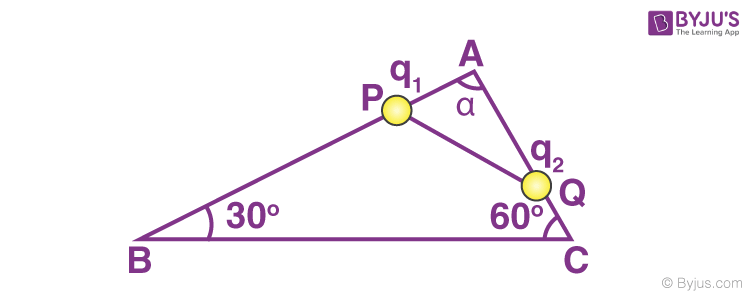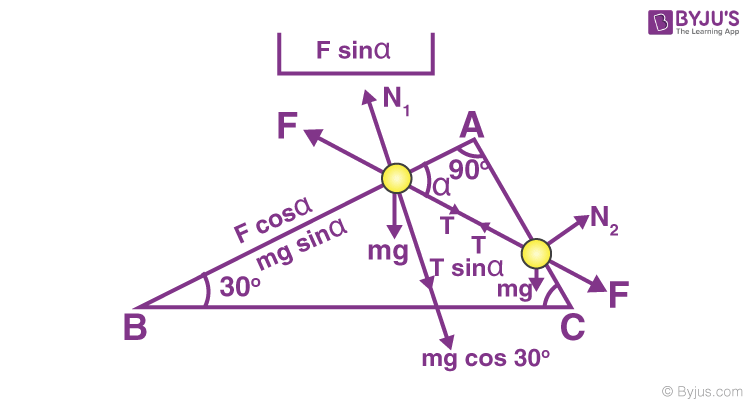Win up to 100% scholarship on Aakash BYJU'S JEE/NEET courses with ABNAT Win up to 100% scholarship on Aakash BYJU'S JEE/NEET courses with ABNAT

# Electric Field Intensity - Motion of Charged Particle in Electric Field

## What Is Electric Field Intensity?

The space around an electric charge in which its influence can be felt is known as the electric field. The electric field intensity at a point is the force experienced by a unit of positive charge placed at that point.

• Electric field intensity is a vector quantity.
• It is denoted by ‘E’.
• Formula: Electric field = F/q.
• Unit of E is NC-1 or Vm-1.

The electric field intensity due to a positive charge is always directed away from the charge, and the intensity due to a negative charge is always directed towards the charge.

Due to a point charge q, the intensity of the electric field at a point d units away from it is given by the expression:

Electric Field Intensity (E) = q/[4πεd2] NC-1

The intensity of the electric field at any point due to a number of charges is equal to the vector sum of the intensities produced by the separate charges.

## Force Experienced by a Charge in an Electric Field

The force experienced by a charge in an electric field is given by

$$\begin{array}{l}\vec{F}=Q\vec{E}\end{array}$$
, where E is the electric field intensity.

### Special Cases:

• If Q is a positive charge,
$$\begin{array}{l}\text{the force}\ \vec{F}\ \text{acts in the direction of}\ \vec{E}.\end{array}$$
Acceleration a = F/m = QE/m.
• If Q is a negative charge, the force acts in a direction opposite to
$$\begin{array}{l}\vec{E}\end{array}$$
. Acceleration a = F/m = – QE/m

A charge in an electric field experiences a force, whether it is at rest or moving. The electric force is independent of the mass and velocity of the charged particle, it depends upon the charge.

## Motion of Charged Particle in an Electric Field

If a charged particle of charge Q is placed in an electric field of strength E, the force experienced by the charged particle = EQ.

• The acceleration of the charged particle in the electric field, a = EQ/m.
• The velocity of the charged particle after time t is = (EQ/m)t if the initial velocity is zero.
• The distance travelled by the charged particle is S = (1/2) × at2 = 1/2(EQ/m) × t2 if the initial velocity is zero.

### The Trajectory of Particle in an Electric Field

When a charged particle is projected into a uniform electric field with some velocity perpendicular to the field, the path traced by it is a parabola. The trajectory of a charged particle projected in a different direction from the direction of a uniform electric field is a parabola.

• When a charged particle of mass m and charge Q remains suspended in a vertical electric field, then mg = EQ.
• When a charged particle of mass m and charge Q remains suspended in an electric field, and the number of fundamental changes on the charged particle is n, then mg = E(ne), thus n = mg/Ee.

### Pendulum Oscillating in a Uniform Electric Field

The bob of a simple pendulum is given a +ve charge, and it is made to oscillate in a vertically upward electric field. Then the time period of oscillation is,

Time Period (T) = 2π × √[l/(g – EQ/m)]

In the above case, if the bob is given a -ve charge, then the time period is given by,

Time Period (T) = 2π × √[l/(g + EQ/m)].

### Projectile Motion in an Electric Field

A charged particle is projected with an initial velocity of u and charges Q, making an angle to the horizontal in an electric field directed vertically upwards, then,

1. Time of flight = 2u sin θ/[g ± EQ/m]

2. Maximum height = u2 sin2 θ/[g ± EQ/m]

3. Range = u2 sin 2θ/[g ± EQ/m]

## Properties of Charged Particle in an Electric Field

1. The density of the electric field inside a charged hollow conducting sphere is zero.

2. A sphere is given a charge of ‘Q’ and is suspended in a horizontal electric field. The angle made by the string with the vertical is, θ = Tan-1 (EQ/mg).

3. The tension in the string is √(EQ2 + mg2).

4. A bob carrying a +ve charge is suspended by a silk thread in a vertically upward electric field, then the tension in the string is, T = mg – EQ.

5. If the bob carries a -ve charge, the tension in the string is, T = mg + EQ.

### Force Experienced by Electron and Proton

A proton and an electron in the same electric field experience forces of the same magnitude but in opposite directions. The force on the proton is accelerating force, whereas the force on the electron is retarding force. If the proton and electron are initially moving in the direction of the electric field,

Acceleration of proton/retardation of electron = mass of an electron/mass of a proton.

## Solved Examples

Question: A rigid insulated wireframe, in the form of a right-angled triangle ABC, is set in a vertical plane, as shown in the figure. Two beads of equal masses m and carrying charges q1 and q2 are connected by a cord of length L and can slide without friction on the wires. Considering the case when the beads are stationary, determine,

1. The angle α (∠APQ)
2. The tension in the cord
3. The normal reactions on the beads
4. If the cords are cut now, what are the values of the charges for which the beads continue to remain stationary?Solution:

1. The forces acting on each bead are,

• mg (downward)
• Tension
• Electric force
• Normal reactions.

The directions of the forces are shown in the figure:Equating the forces along and perpendicular to AB and AC for the equilibrium of the beads,

T cos α = F cos α + mg sin 300 . . . . (1)

F sin α + N1 = mg cos 300 + T sin α . . . . . (2)

T sin α = F sin α + mg cos 30. . . . . . (3)

N2 + F cos α = T cos α + mg cos 600 . . . . . (4)

From equations (1) and (3),

(T – F) cos α = mg sin 300

and (T – F) sin α = mg cos 300

Dividing, cot α = tan 300 = cot 600

Therefore, α = 600.

2. Substituting the value of α in (1) and noting that

$$\begin{array}{l}F = \frac{1}{4\pi {{\varepsilon }_{0}}}\;\;\frac{{{q}_{1}}{{q}_{2}}}{{{\ell }^{2}}}\end{array}$$
$$\begin{array}{l}T\ cos\ 60^\circ = \frac{1}{4\pi {{\varepsilon }_{0}}}\;\;\frac{{{q}_{1}}{{q}_{2}}}{{{\ell }^{2}}}\end{array}$$
cos 60º + mg sin 60º

or

$$\begin{array}{l}T = \frac{1}{4\pi {{\varepsilon }_{0}}}\;\;\frac{{{q}_{1}}{{q}_{2}}}{{{\ell }^{2}}} + mg\end{array}$$

3. From (2), N1 = mg cos 30º + (T – F) sin 60º

⇒ N1 = mg

$$\begin{array}{l}\frac{\sqrt{3}}{2} + mg . \frac{\sqrt{3}}{2}= \sqrt{3} mg\end{array}$$

From (4), N2 = (T – F) cos 600 + mg cos 600

= mg × 1/2 + mg × 1/2 = mg.

4. When the cord is cut, T = 0, then from (1)

0 = F cos 600 + mg sin 300

or F + mg = 0

This result is on the assumption that q1 and q2 are of the same sign, so it was taken that there was a force of repulsion.

Since mg is fixed in direction, its sign cannot be reversed, but the sign of F can be reversed because if q1 and q2 are of opposite sign, F will change its sign from + to -.

Let q1 and q2 be of the opposite sign, then,

-F + mg = 0 is the condition for equilibrium.

or

$$\begin{array}{l}\frac{1}{4\pi {{\varepsilon }_{0}}}\;\;\frac{{{q}_{1}}{{q}_{2}}}{{{\ell }^{2}}} = mg\end{array}$$

or

$$\begin{array}{l}q_1q_2 = 4\pi\varepsilon _0mg\end{array}$$

## Frequently Asked Questions on Electric Field Intensity

Q1

### The test charge used to measure the electric field intensity at a point should be infinitesimally small. Why?

The test charge used must be infinitesimally small so that it will not produce a field of its own. The actual value of the electric field intensity will get altered because of the field produced by the test charge.

Q2

### Is electric field intensity a scalar or a vector quantity?

Electric field intensity is a vector quantity.

Q3

### Define electric intensity at a point in an electric field.

Electric intensity at a point is the force on a unit positive charge at that point.

Test Your Knowledge On Electric Field Intensity!